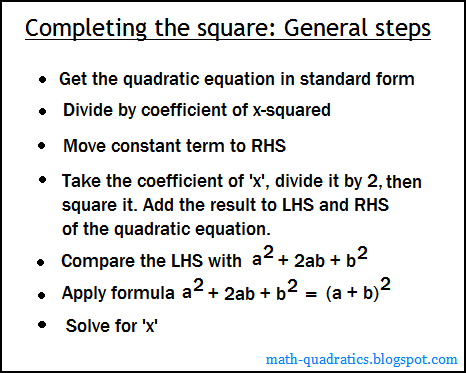# Unit 1!

` On this page I will be explaining Completing The Square,  which is a technique for finding the x values of a quadratic polynomial.`

#### Step 1: Write the equation

x^2 + 12x + 32 = 0

x^2 + 12x = -32

#### Step 3: Add (b/2)^2 to both sides.

b = 12 so 12/2 = 6, 6^2 = 36. Our equation will now look like: x^2 + 12x + 36 = 4

#### Step 4: Factor left side of the equation.

x^2 + 12x + 36 = (x+6)(x+6)

(x+6)^2 = 4

#### Step 6: Find the square root of both sides.

We do this to simplify the equations so we're able to use our properties to find x.

x+-6 = +-4

#### Step 7: Find the x's.

x = -4, -8